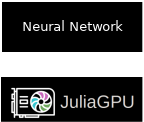# GPU-accelerated Neural Networks with Julia and oneAPI

### November 28, '21Hello again, dear readers! This time it’s going to be a quick post about how to get hardware acceleration in Julia for scientific computing, using neural network execution as an example. This is going to be specifically for Intel graphics cards (there’s a lot of Intel GPU stuff in this blog, huh? 😁).

I also have added support for typing emoji so maybe I’ll use more emoji now? 🥳

Julia is a good language for scientific computing for several reasons, in my opinion:

• good support for unicode math notations (easily writing stuff like ẏ for the derivative of y is a powerful tool when your audience is used to math notation)
• excellent math library (I am usually doing linear algebra stuff, so that’s what I’m talking about here)
• fast: when you are the number crunching language, you can’t just say “use a C library to do the number crunching”
• pretty good packaging: most things don’t require complex installation and work in the same way across platforms, the only drawback is that compiling sometimes takes a while

## On Julia’s Documentation

One point however that’s still quite lacking is the documentation, you can easily find corners of the language that are not well documented or simple idioms that you seem to not be able to find. In those cases, reading some existing projects is always the best source of information to get you back on track.

# oneAPI

As explained in the oneAPI.jl intro blogpost (be sure to also check the oneAPI.jl homepage and the actual Intel documentation about oneAPI), you can quickly download everything with a simple command (see what I was talking about when I said “pretty good packaging”?):

pkg> add oneAPI


📒 To check how to enter the pkg> prompt read the Pkg reference @ Julia docs

After everything is downloaded, we can simply start to type a new Julia program with:

using oneAPI


and then use the oneArray API to get things ACCELERATED!

# Feed Forward Neural Network

Lets start out by defining what the key data structure for neural networks is: a matrix of real numbers.

const RealMat = AbstractArray{<:Real}


In this case we set the type to AbstractArray so later on we can construct a neural network for different types of arrays.

Now, lets make define data structures useful in writing clearer code to handle the computation of neuron layers:

const LayerInput = RealMat

struct Layer
weights::RealMat
biases::RealMat
end

struct LayerOutput
# The value of the layer output before running the activation function over
# each element
partial::RealMat
# And after running ϕ (activation function) after each element
final::RealMat
end


Once that’s done we have the entire structure of a neural network quite well defined. We can do the activation function next:

ϕ(x) = max(x, 0)


Here we are using the ReLU function.

Okay, great work so far, but what about actually computing the output value of the nn? 😬 That comes now:

# Compute the outputs for each layer, given the input for the initial layer and
# the actual data (weights and biases) for each layer
function feed_forward(input::LayerInput, network::Vector{Layer})::Vector{LayerOutput}
# If there's nothing in the nework, then there should be nothing in the
# output too... 🤔
isempty(network) && return []

# We are calling feed_forward recursively, so first(network) is always the
# "current" element we want to calculate with
layer = first(network)

partial_output = input * layer.weights + layer.biases
output = LayerOutput(partial_output, ϕ.(partial_output))

if length(network) == 1
[output]
else
# Concatenate the current input with the output for the next layers
[output; feed_forward(output.final, network[2:end])]
end
end


And we are done! The only step that’s now missing is actually computing the value for some network. We are going to do this now ✨

gpu_network = oneArray(
Layer(oneArray(rand(4096, 4096)), oneArray(rand(1, 4096))),
Layer(oneArray(rand(4096, 4096)), oneArray(rand(1, 4096))),
Layer(oneArray(rand(4096, 1)), oneArray(rand(1, 1))),
)

gpu_input = oneArray(rand(1, 4096))

println(last(feed_forward(gpu_input, gpu_network)).final)


This is where the actual magic happens. By using oneArray here to construct the layers, we are making sure that when we call the multiply, map and sum functions in feed_forward, they are actually going to call routines which transfer the memory to the GPU and compute those operations on the GPU.

Pretty simple when you think about it right? It’s one of the best APIs for GPU programming I have ever seen, actually!

# Future Work

In this example there are still some things we probably can optimize, specially around how we are getting the output: in a growable vector on the main memory. That means we are probably pausing the GPU a lot to copy data back.

If instead we did all operations writing back to GPU memory and copying only at the end, we would probably see better performance. Those are things to play around, however. This post was just an introduction for you to see how easy it to turn your GPU into a heater with Julia!

See you all! 💕